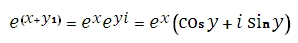# SQL Server IMEXP function

Updated: 27 August 2010
Use IMEXP to return the exponential of a complex number in x + yi or x + yj text format. The exponential is computed as:Syntax
SELECT [wctEngineering].[wct].[IMEXP] (
<@inumber, nvarchar(4000),>)
Arguments
@inumber
is the value at which to evaluate the function. @inumber is an expression of type varchar or of a type that can be implicitly converted to varchar and must be in the format x + yi or x + yj, where x is a real number that can be converted to float and yi (or yj) is an imaginary number, where the y part can be converted to float.
Return Types
varchar(4000)
Remarks
·         Use COMPLEX to convert real and imaginary coefficients into a complex number
Examples

select
wct.IMEXP('7+24i')

This produces the following result
-------------------------
465.168764555019-993.087259331794i

(1 row(s) affected)

### SupportCopyright 2008-2021 Westclintech LLC         Privacy Policy        Terms of Service